# Dealing with Variation in Cold Junction Temperatures

Thin film thermopile detectors require a reference junction temperature measurement or a constant temperature sink for their reference junction. It is possible to measure the detector reference junction temperature by connecting a temperature transducer, such as signal diode, thermistor bead and integrated circuit, to the detector case. However, these transducers require power for operation, thus leading to self-heating.

As a result, instrument designers have to be careful as the introduction of thermal transient from the self-heating of the temperature transducer may upset the delicate thermal balance between the reference and active junctions of the thermopile detector. Dexter Research considered this fact in the design of their temperature compensated DC radiometer.

## Temperature Compensating Network

The implementation of a temperature compensating network involves three major tasks:

• A temperature transducer has to be attached to detect the reference junction of the thermopile
• A circuit has to be designed to integrate the detector voltage with the compensating voltage
• The voltages have to be scaled up to a fixed calibration scheme

Heat is predominantly transferred from and to the reference junctions of the thermopile via the TO-5 header leads and the header itself. A glass to metal bond sealing the leads to the header thermally isolates the leads from the TO-5 case.

The ideal site to connect the temperature transducer to measure reference junction temperature is between these leads. In some cases, the self heating of the temperature transducer generates a signal several hundred times higher than the signal to be detected.

Figure 1 shows the proper attachment of a temperature transducer.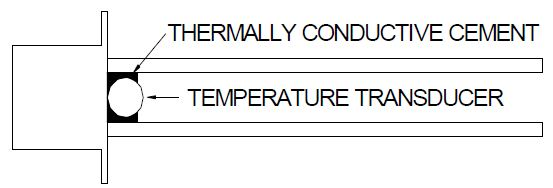Figure 1. Temperature transducer attached to detector leads

The key features include:

• The transducer is thermally connected to the detector leads and by lead conduction to the detector reference junctions
• The transducer is thermally connected to the detector header, thus damping thermal transients
• The detector holder conducts away the self heating of the transducer (not shown in Figure 1)

The YSI-44201 Thermistor will be the cornerstone of the circuit design. The fundamental principles are similar for the other devices, but there would be changes in the circuit details. Figure 2 presents a circuit involving a Thermistor bead B1 connected to a detector model 1M.

The detector voltage is amplified by A1 and the instrument calibration is performed using R3.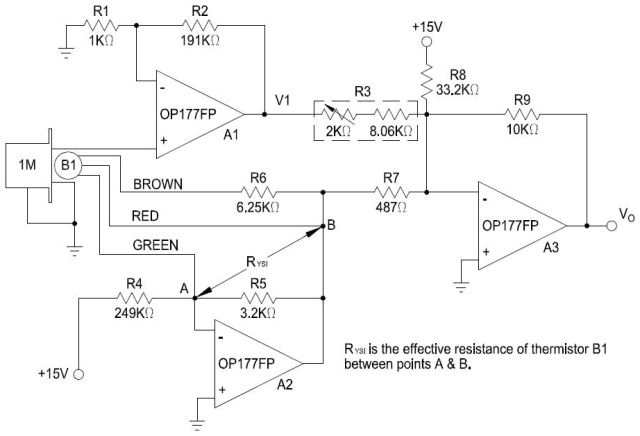Figure 2. Radiometer circuit with temperature compensation

The voltage V1 is expressed in Equation 1: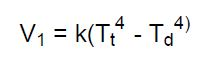Where,

k = systems constant, which includes detector parameters, gain of A1, and optical system

Tt = absolute target temperature in Kelvin.

Td = absolute target temperature in Kelvin.

If the inputs to R7 and R8 are zero, then A3 has an output of (Equation 2):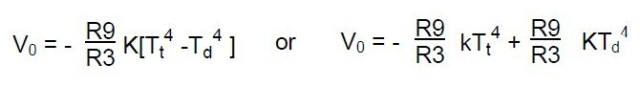This shows the need of subtracting a voltage equal to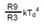from V0 to compensate for the detector reference junction temperature.

The voltage from A2 is (Equation 3):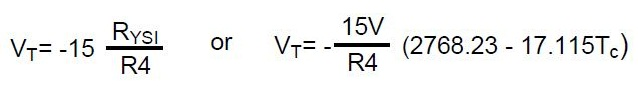Where,

RYSI = 2768.23 - 17.115Tc

Tc = Detector case temperature in °C

The decimal numerical values are obtained from the YSI-44201 data sheet with values for R5 & R6 as presented. A3 adds this voltage, the detector voltage and the reference voltage at the top of R8. The final output voltage of A3 is expressed in Equation 4: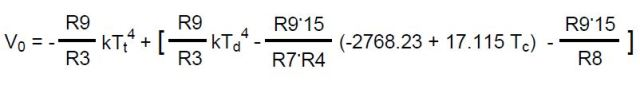When the bracketed term is “zero” the radiometer is compensated and the voltage from A3 is expressed in Equation 5: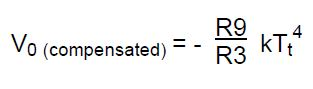The resulting value will form the basis of the calibration scheme. The result of that design is summarized in Table 1.

Table 1. Un-amplified detector voltage for a simple radiometer w/ ambient temperature = 20°C

Temperature (Tt) Target Detector Voltage (Vdet) Compensated Voltage (V0)
K °C mV mV
273 0 -1.62 5.18
298 25 0.46 7.35
323 50 3.14 10.01
373 100 10.71 18.04
473 200 38.14 46.64

For this design, a target temperature of 200°C will be allowed to provide 10V at the output of A3. The gain of A1 can be determined as G = 10/.04664 = 214.41. Since the variation of the responsivity between detectors is ±10%, G will be approximated with the gain of A1 and set using available resistor values, R1 = 1K and R2= 191K, yielding the gain of A1 as 192.

During instrument calibration, the ratio of R9/R3 will be adjusted to provide the desired system gain to give an output of 10V at 200°C.

The instrument constant will be then determined when V0=10V at Tt =473K using Equation 5: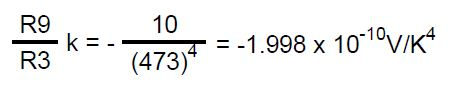The radiometer is assumed to be used in ambient temperature range of 0-50°C. The required compensating voltage can be plotted using the previously calculated ambient temperature range and instrument constant (Figure 3) to provide an accurate output voltage in proportion to the target temperature.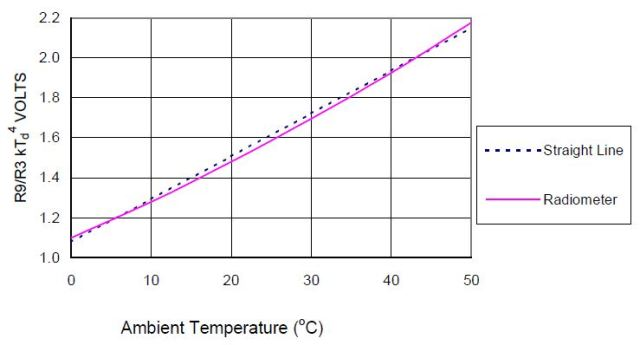Figure 3. Required compensating voltage from equation 4 verses ambient temperature with a straight line fit.

The dashed line represents the linear voltage fit from the Thermistor (1.08V at 0°C and 2.15V at 50°C). For an equation of Vc = mTc + b (Equations 6 and 7):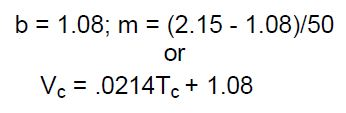Equating like coefficients of the bracketed portion of Equation 4 and Equation 7, Equation 8 can be obtained: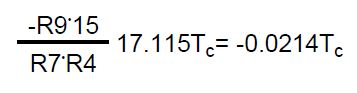R4 is set equal to 249KΩ to maintain thermistor self heating low. Applying Equation 8 and solving utilizing standard resistor values, R9 is set equal to 10KΩ,yielding R7 = 482Ω. Selecting the closest 1% resistor, R7 is set equal to 487Ω (Equation 9):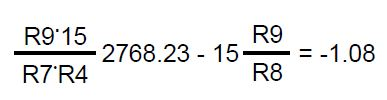Using Equation 9, R8 is determined to be33.3KΩ. Selecting the closest 1% resistor, R8 is equal to 33.2KΩ. The resulting values of R4, R7, R8, and R9 have been substituted into the bracketed terms of Equation 4.

Using Equation 6, Equation 10 will be derived as follows: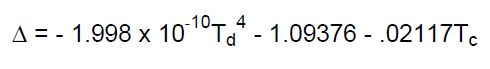Figure 4 presents a plot of voltage error of equation 10.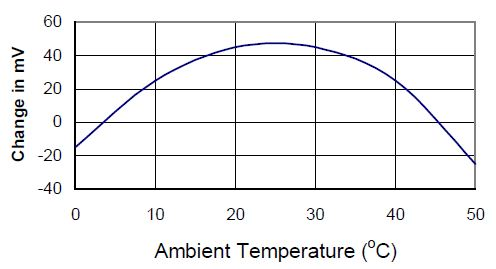Figure 4. Error in compensating voltage caused by linear fit of 4th Power law.

## Conclusion

Simple circuitry and 1% resistors were employed in conjunction with a bead thermistor. The fundamental principle may be applied utilizing other temperature transducers.This information has been sourced, reviewed and adapted from materials provided by Dexter Research.

## Citations

• APA

Dexter Research Center, Inc.. (2019, December 24). Dealing with Variation in Cold Junction Temperatures. AZoSensors. Retrieved on November 28, 2020 from https://www.azosensors.com/article.aspx?ArticleID=597.

• MLA

Dexter Research Center, Inc.. "Dealing with Variation in Cold Junction Temperatures". AZoSensors. 28 November 2020. <https://www.azosensors.com/article.aspx?ArticleID=597>.

• Chicago

Dexter Research Center, Inc.. "Dealing with Variation in Cold Junction Temperatures". AZoSensors. https://www.azosensors.com/article.aspx?ArticleID=597. (accessed November 28, 2020).

• Harvard

Dexter Research Center, Inc.. 2019. Dealing with Variation in Cold Junction Temperatures. AZoSensors, viewed 28 November 2020, https://www.azosensors.com/article.aspx?ArticleID=597.